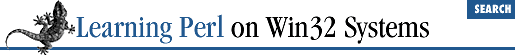home | O'Reilly's CD bookshelfs | FreeBSD | Linux | Cisco | Cisco Exam4.2 The if/unless Statement

Next up in order of complexity is the if statement. This construct takes a control expression (evaluated for its truth) and a block. It may optionally have an else followed by a block as well. In other words, it looks like this:

if (

some_expression

) {

true_statement_1

;

true_statement_2

;

true_statement_3

;
}

else {

false_statement_1

;

false_statement_2

;

false_statement_3

;
}

(If you're a C or Java hacker, you should note that the curly braces are required . This eliminates the need for a " confusing dangling else" rule.)

During execution, Perl evaluates the control expression. If the expression is true, the first block (the true_statement statements above) is executed. If the expression is false, the second block (the false_statement statements above) is executed instead.

But what constitutes true and false? In Perl, the rules are slightly weird, but they give you the expected results. The control expression is evaluated for a string value in scalar context (if it's already a string, no change is made; but if it's a number, it is converted to a string[ 1 ] ). If this string is either the empty string (with a length of zero) or a string consisting of the single character 0 (the digit zero), then the value of the expression is false. Anything else is true automatically. Why such funny rules? Because they facilitate branching on an emptyish versus nonempty string, as well as on a zero versus nonzero number, without having to create two versions of interpreting true and false values. Here are some examples of true and false interpretations:

 Internally, this isn't quite true. But it acts as if this is what it does.

0       # converts to "0", so false
1-1     # computes to 0, then converts to "0", so false
1       # converts to "1", so true
""      # empty string, so false
"1"     # not "" or "0", so true
"00"    # not "" or "0", so true (this one is weird, watch out)
"0.000" # also true for the same reason and warning
undef   # evaluates to "", so false

Practically speaking, interpretation of values as true or false is fairly intuitive. Don't let us scare you.

Here's an example of a complete if statement:

print "how old are you? ";
\$a = <STDIN>;
chomp(\$a);
if (\$a < 18) {
print "So, you're not old enough to vote, eh?\n";
} else {
print "Old enough!  Cool!  So go vote!\n";
\$voter++; # count the voters for later
}

You can omit the else block, leaving just a then part, as in:

print "how old are you? ";
\$a = <STDIN>;
chomp(\$a);
if (\$a < 18) {
print "So, you
'
re not old enough to vote, eh?\n";
}

Sometimes, you want to leave off the then part and have just an else part, because saying "do that if this is false," is more natural than saying "do that if not this is true." Perl handles this case with the unless variation:

print "how old are you? ";
\$a = <STDIN>;
chomp(\$a);
unless (\$a < 18) {
print "Old enough!  Cool!  So go vote!\n";
\$voter++;
}

Replacing if with unless is in effect saying, "If the control expression is false, do..." (An unless can also have an else , just like an if .)

If you have more than two possible choices, add an elsif branch to the if statement, like so:

if (

some_expression_one

) {

one_true_statement_1

;

one_true_statement_2

;

one_true_statement_3

;
}

elsif (

some_expression_two

) {

two_true_statement_1

;

two_true_statement_2

;

two_true_statement_3

;
} elsif (

some_expression_three

) {

three_true_statement_1

;

three_true_statement_2

;

three_true_statement_3

;
} else {

all_false_statement_1

;

all_false_statement_2

;

all_false_statement_3

;
}

Each expression (here, some_expression_one , some_expression_two , and some_expression_three ) is computed in turn. If an expression is true, the corresponding branch is executed, and all remaining control expressions and corresponding statement blocks are skipped. If all expressions are false, the else branch is executed (if there is one). You don't have to have an else block, but having one is always a good idea. You may have as many elsif branches as you wish.4.1 Statement Blocks4.3 The while/until Statement